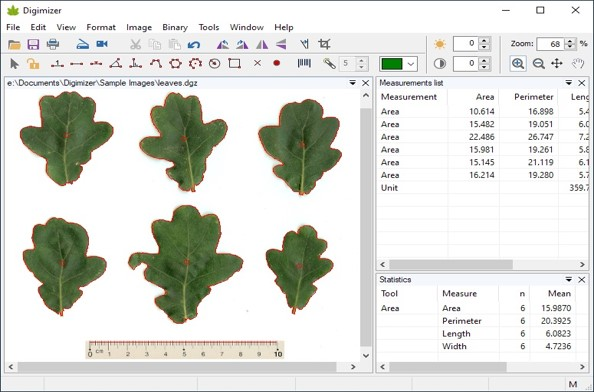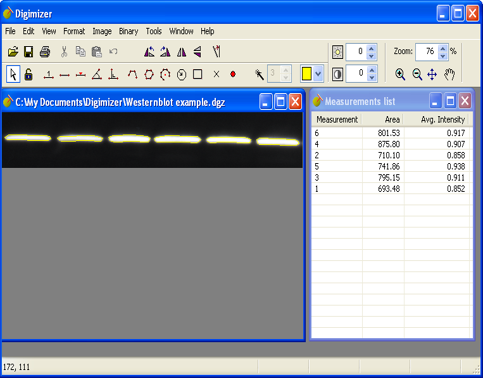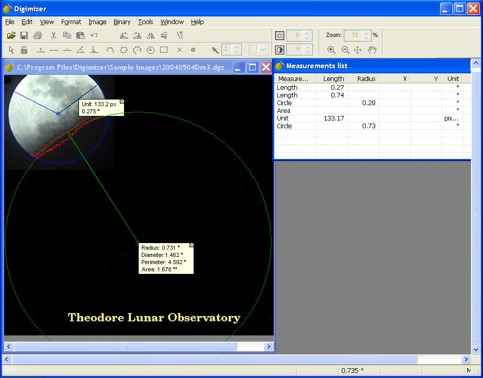Digimizer | 医学图像分析软件

Digimizer是一款易于使用且灵活的图像测量分析软件，可以对图像内容进行手动测量，根据测量对象的特点进行自动目标检测! 图像可以是X光图片、显微照片等，支持JPG、GIF、TIFF、BMP、PNG、WMF和EMF等多种图片格式! 图像还可以进行旋转、反转、拉伸等处理和图像明暗度、比对等进行调节! 可以应用多个过滤器。当前Digimizer的新版本为Version 5.4.7。1. 测量分析

2. 自动识别

3. 多种形式

4. 支持格式

5. 处理便捷

• 打开，保存和打印图像文件

• 从扫描仪获取图像

• 从实时视频流中抓取图像

• 支持的文件格式：DGZ（本机Digimizer文件格式），JPG，GIF，TIFF，BMP，PNG，WMF，EMF和DICOM文件。

• 调整

• 作物

• 旋转，翻转

• 放大

• 调整比对数值和亮度

• 比对数值自动修复

• 拉伸直方图

• 背景校正

• 去斑

• 转换为灰度

• 转换为颜色

• 倒置

• 削尖

• 滤波器：浮雕，算术平均滤波器，几何平均滤波器，谐波平均滤波器，中值滤波器，大滤波器，小滤波器，中点滤波器，Yp均值滤波器

Digimizer允许：

• 定义计量单位

• 测量线段或路径的距离和长度

• 衡量周长和面积

• 测量角度

• 找到线段的中间位置

• 找到中心并计算圆形物体的特征

• 垂直线上的测量

• 测量圆和矩形

• 测量物体的平均强度

• 标记和计数对象

• 适合线

• 检测和解码条形码和QR码

• 二值化

• 选项：叠加二进制图像，隐藏二进制图层

• 形态学操作：扩张，腐蚀，开放，关闭

• 降噪

• 分析对象：通过测量周长和面积进行物体检测

• 打开并保存测量列表

• 打印测量列表

• 导出为Excel电子表格文件或MedCalc文件进行统计分析

“综合统计”窗口显示“测量”列表中测量的统计数据（n，平均值，标准差等）。

• 打印统计信息

Miscelaneous

• 用户定义的脚本允许重复性任务的自动化Digimizer由于其演算速度快，计算机可用于准确的表达式，测量和设计。并且可以使用多种应用程序，它配备着一套功能强大的测量工具，可以自动收集和存储数据。• Windows 10

• Windows Vista，Windows 7或Windows 8

• 1024MB的内存

• 硬盘上有20 MB可用空间

【英文介绍】

Image manipulation

• Resize

• Crop

• Rotate, flip

• Zoom

• Contrast auto fix

• Stretch histogram

• Background correction

• Despeckle

• Convert to grayscale

• Convert to color

• Invert

• Negative

• Sharpen

• Filters: Emboss, Arithmetic Mean Filter, Geometric Mean Filter, Harmonic Mean Filter, Median Filter, Maximum Filter, Minimum Filter, Midpoint Filter, Yp Mean Filter

Manual measurements

Digimizer allows to:

• define unit of measurement

• measure distances and lengths of line segments or paths

• measure perimeters and areas

• measure angles

• locate middle of line segment

• find center and calculate characteristics of circular objects

• measurements on perpendicular lines

• measure circles and rectangles

• measure average intensity of objects

• mark and count objects

• fit line

• detect and decode bar codes and QR codes

• Units of measurements: kilometer (km), hectometer (hm), decameter (dam), meter (m), centimeter (cm), millimeter (mm), micrometer (µm), nanometer (nm), picometer (pm), femtometer (fm), attometer (am), inch (in), foot (ft), yard (yd), mile (mi), degrees (°) or pixels.

Image analysis

• Binarization

• Options: overlay binary image, hide binary layer

• Morphological operations: Dilate, Erode, Open, Close

• Noise reduction

• Analyze objects: object detection with measurement of perimeter and area

Measurements list

The measurements list displays the measurements in the current image, but can also include measurements of several images.

• Open & save Measurements list

• Print Measurements list

• Export as Excel spreadsheet file, or MedCalc file for statistical analysis

Statistics

The integrated statistics window displays statistics (n, mean, SD, minimum and maximum) of the measurements in the Measurements list.

• Print statistics

Miscelaneous

• User-defined scripts allow automation of repetitive tasks